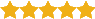<MyRusakov.ru />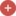Регистрация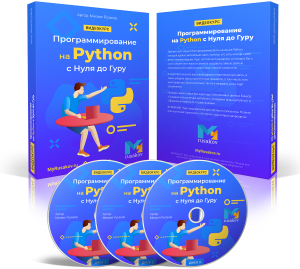Программирование на Python с Нуля до Гуру

Данный курс научит Вас программировать на языке Python, который крайне желательно знать любому, кто хоть иногда имеет дело с компьютерами. Курс состоит из 6 разделов, в которых Вы с нуля освоите этот язык и сможете создавать самые разные программы для самых разных задач любой сложности.

К курсу прилагается множество упражнений и все исходники из уроков.

Наконец, Вы получите ещё несколько бонусов: "Создание калькулятора на Python", "Создание игры на Python" и "Правильная работа со справочником".

Подробнее
Уроки и статьи
Подписка

Подписавшись по E-mail, Вы будете получать уведомления о новых статьях.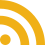Добавляйтесь ко мне в друзья ВКонтакте! Отзывы о сайте и обо мне оставляйте в моей группе.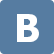Опрос

Каким движком Вы предпочитаете пользоваться?

Форум сайта MyRusakov.ru

php игра
14.05.2013 22:22:50 php игра Сообщение #1arman

Новичок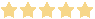Дата регистрации:
01.03.2013 15:29:05

Сообщений: 7

Попытался сделать игру, которая угадывает квадратный корень, наподобие угадывания чисел, но она продолжает угадывать числа вместо нахождения корня.
Код:
<?php
\$message = "Guess the number in the range from 0 to 10000!";
if (isset(\$_POST["guess"])) {
\$number = \$_POST["number"];
\$hidden_number = \$_POST["hidden_number"];
\$s = sqrt(\$hidden_number);
if (\$number < \$s) {
\$message = "Your number is less than \$number";
}
elseif (\$number > \$s) {
\$message = "Your number is more than \$number";
}
elseif (\$number == \$s) {
\$message = "Congratulations! You won! <br/> Guess new number!";
\$hidden_number = rand(0, 10000);
}
}
else {
\$number = 0;
\$hidden_number = rand(0, 10000);
}
?>
<html>
<title>Guess the number!</title>
<body>
<h2><?php echo \$message; ?></h2>
<form method = "post">
<input type = "text" name = "number" value = "<?php echo \$number ?>" />
<input type = "submit" name = "guess" value = "Guess!" />
<input type = "hidden" name = "hidden_number" value = "<?php echo \$s ?>" />
</form>
</body>
</html>
14.05.2013 23:14:48 php игра Сообщение #2